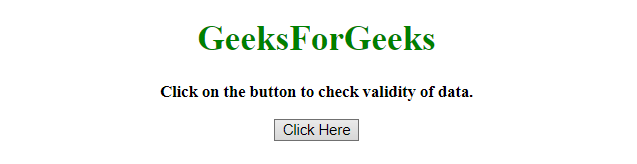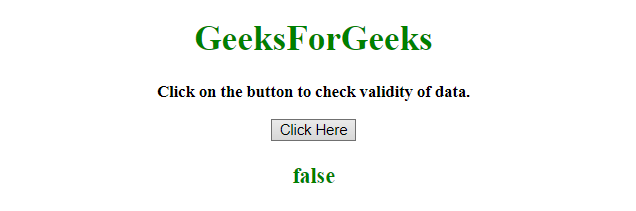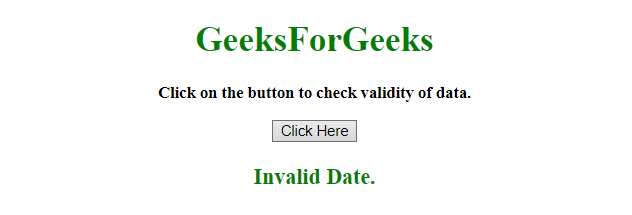# How to check a date is valid or not using JavaScript ?

Given a date object and the task is to check the given date is valid or not using JavaScript. There are two methods to solve this problem which are discussed below:

Approach 1:

• Store the date object in a variable.
• If the date is valid then the getTime() will always be equal to itself.
• If the date is Invalid then the getTime() will return NaN which is not equal to itself.
• The isValid() function is used to check the getTime() method is equal to itself or not.

Example 1: This example implements the above approach.

 `  ` `<``html``>  ` ` `  `<``head``>  ` `    ``<``title``>  ` `        ``How to check a date is valid ` `        ``or not using JavaScript ? ` `    `` ` `  ` ` `  `<``body` `style` `= ``"text-align:center;"``>  ` `     `  `    ``<``h1` `style` `= ``"color:green;"` `>  ` `        ``GeeksForGeeks  ` `    `` ` `     `  `    ``<``p` `id` `= ``"GFG_UP"` `style` `= ` `        ``"font-size: 15px; font-weight: bold;"``> ` `    `` ` `     `  `    ``<``button` `onclick` `= ``"GFG_Fun()"``> ` `        ``Click Here ` `    `` ` `     `  `    ``<``p` `id` `= ``"GFG_DOWN"` `style` `= ` `        ``"color:green; font-size: 20px; font-weight: bold;"``> ` `    `` ` `     `  `    ``<``script``> ` `        ``var up = document.getElementById('GFG_UP');  ` `        ``var down = document.getElementById('GFG_DOWN');  ` `        ``var d = new Date("2012/2/30"); ` `         `  `        ``up.innerHTML = "Click on the button to check" ` `                    ``+ " validity of data.<``br``>"; ` `         `  `        ``Date.prototype.isValid = function () { ` `             `  `            ``// If the date object is invalid it ` `            ``// will return 'NaN' on getTime()  ` `            ``// and NaN is never equal to itself. ` `            ``return this.getTime() === this.getTime(); ` `        ``}; ` `         `  `        ``function GFG_Fun() { ` `            ``down.innerHTML = d.isValid(); ` `        ``}  ` `    ``  ` `  ` ` `  ` `

Output:

• Before clicking on the button:• After clicking on the button:Approach 2:

• Store the date object into a variable d.
• Check if the variable d is created by Date object or not by using Object.prototype.toString.call(d) method.
• If the date is valid then the getTime() method will always be equal to itself.
• If the date is Invalid then the getTime() method will return NaN which is not equal to itself.
• In this example, isValid() method is checking if the getTime() is equal to itself or not.

Example 2: This example implements the above approach.

 `  ` `<``html``>  ` ` `  `<``head``>  ` `    ``<``title``>  ` `        ``How to check a date is valid ` `        ``or not using JavaScript ? ` `    `` ` `  ` ` `  `<``body` `style` `= ``"text-align:center;"``>  ` `     `  `    ``<``h1` `style` `= ``"color:green;"` `>  ` `        ``GeeksForGeeks  ` `    `` ` `     `  `    ``<``p` `id` `= ``"GFG_UP"` `style` `= ` `        ``"font-size: 15px; font-weight: bold;"``> ` `    `` ` `     `  `    ``<``button` `onclick` `= ``"GFG_Fun()"``> ` `        ``Click Here ` `    `` ` `     `  `    ``<``p` `id` `= ``"GFG_DOWN"` `style` `= ` `        ``"color:green; font-size: 20px; font-weight: bold;"``> ` `    `` ` `     `  `    ``<``script``> ` `        ``var up = document.getElementById('GFG_UP');  ` `        ``var down = document.getElementById('GFG_DOWN');  ` `        ``var d = new Date("This is not date."); ` `         `  `        ``up.innerHTML = "Click on the button to check " ` `                    ``+ "validity of data.<``br``>"; ` `         `  `        ``function GFG_Fun() { ` `         `  `            ``if (Object.prototype.toString.call(d) ` `                                    ``=== "[object Date]") ` `            ``{ ` `                ``if (isNaN(d.getTime())) {  ` `                    ``down.innerHTML = "Invalid Date."; ` `                ``} ` `                ``else { ` `                    ``down.innerHTML = "Valid Date."; ` `                ``} ` `            ``} ` `        ``}  ` `    ``  ` `  ` ` `  ` `

Output:

• Before clicking on the button:• After clicking on the button:My Personal Notes arrow_drop_upCheck out this Author's contributed articles.

If you like GeeksforGeeks and would like to contribute, you can also write an article using contribute.geeksforgeeks.org or mail your article to contribute@geeksforgeeks.org. See your article appearing on the GeeksforGeeks main page and help other Geeks.

Please Improve this article if you find anything incorrect by clicking on the "Improve Article" button below.